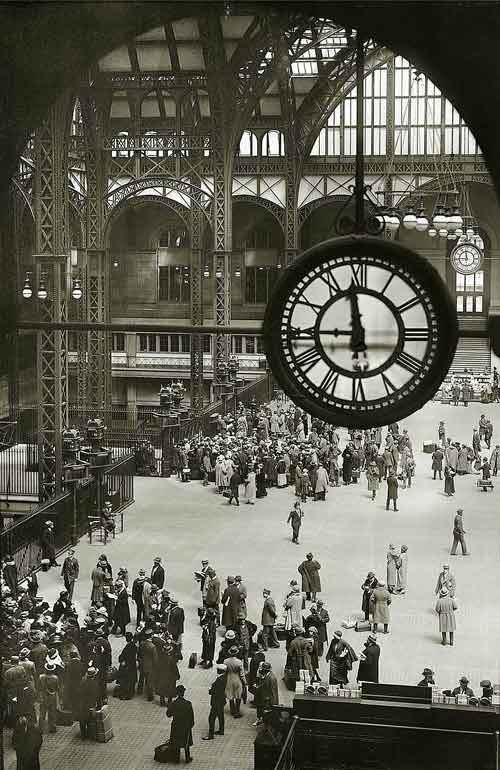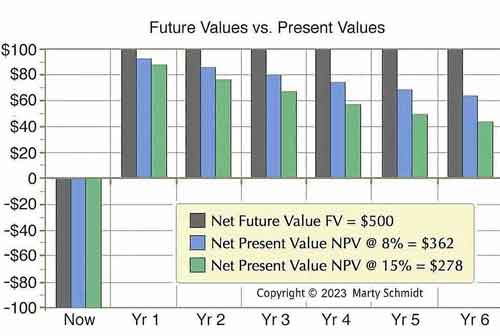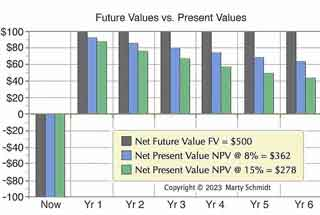## What is Discounted Cash Flow?

The Time Value of Money concept explains why the present value of every transaction depends on the length of time before payment.

Time-value-of-money and discounted cashflow concepts are the cornerstone of modern finance.

When investment projections or business case results extend more than a year into the future, finance professionals invariably want to see cash flow forecasts in two forms—with discounting and without discounting. Financial specialists, that is, want to know the time value of money impact on long-term projections.

### Define Time Value of Money and Discounted Cash Flow

The time-value-of-money concept is the idea that money flowing in or flowing out at some time in the future has less value, today, than an equal amount present or flowing in today.

The discounted cash flow (DCF) calculation is an application of the time value of money concept. DCF finds the value appropriate today for a future cash flow—the present value. The term discounting applies because the DCF present value (PV) is always lower than the cash flow future value (FV).

### Core Concepts: Present Value and Future Value

Two time-value-of-money concepts are central in discounted cash flow analysis:

• Present value (PV) is what the future cash flow is worth today.
• Future value (FV) is the value that flows in or out at the designated time in the future.

A \$100 cash inflow that will arrive two years from now could, for example, have a present value today of about \$95, while its future value is by definition \$100.

• For each cash flow event, the present value is less than the corresponding future value, except for cash flow events occurring today, in which case PV = FV).
• The further into the future before a cash flow event occurs, the more discounting lowers the present value below its future value.
• the total of all of the cash flow present values for the cash flow series, across a time span extending into the future, is the net present value (NPV) of a cash flow streamEveryone in the station is acutely aware of the clock and time—current time, departure time, travel time. Similarly, anyone planning financial transactions must know precisely the calendar time in view—the the investment life, loan life, and the time before funds flow. Discounting and interest calculations quantify the time value of money in each case.  [Photo: Penn Station, New York, 11:45 AM, 1 January, 1924]Everyone in the station is acutely aware of the clock and time—current time, departure time, travel time. Similarly, anyone planning financial transactions must know precisely the calendar time in view—the the investment life, loan life, and the time before funds flow. Discounting and interest calculations quantify the time value of money in each case.  [Photo: Penn Station, New York, 11:45 AM, 1 January, 1924]

DCF is center stage when decision-makers and investors evaluate potential investments, action proposals, or purchases. Looking forward in time, the analyst projects cash inflows and outflows (cash flow streams) the investor can expect from each of these. Other things being equal, analysts will recommend the action or investment with the more substantial cash flow NPV, as the "better business decision."

### Explaining Time Value of Money in Context

Sections below describe and illustrate Discounted Cash Flow and other Time Value of Money terms in context with similar ideas from business analysis, banking, and finance, focusing on four themes:

• First, defining Time Value of Money in Finance, and why this value is real and measurable.
• Second, the meaning of Time Value and Discounting concepts, such as Present Value, Future Value, and Discount Rate.
• Third, example calculations showing how to discount future values to present values in cash flow streams, and how to calculate Net Present Value (NPV).
• Fourth, the role of DCF and NPV in Business Case Analysis and Business Planning.

## Is Time-Value-of-Money Real Value?Is Time-Value Important in Finance?

When first hearing the definition of discounted cash flow, many people understandably react with comments like these: "It sounds like fiction" or "The time value of money cannot refer to real value because DCF does not measure real cash flow" or "Exactly where is the tangible value in time value?" Business professionals begin answering such questions by presenting the idea that value results from having the use of money:

Having the use of money for a time period has a value that is tangible, measurable, and real.

Discounted cash flow (DCF) is one application of this concept, while interest paid for a loan is another. With DCF, the discounting lowers the present value PV of future funds below the future value FV of the funds for at least three reasons:

1. Opportunity.
Funds you have now could (in principle) be invested now, and gain return or interest between the present and the future time. You cannot use now funds that you will not have until a some time in the future.
2. Risk
Funds you have now are not at risk, but funds arriving in the future are uncertain. A familiar proverb states this principle: "A bird in the hand is worth two in the bush."
3. Inflation.
A sum you have today will very likely buy more than an equal amount you will not have until years in future. The buying power of money decreases over time due to inflation.

## Define Your Terms! Present, Future, NPV

What future money is worth today is its present value (PV) and what it will be worth in the future when it finally arrives is, not surprisingly, its future value (FV).

The right to receive a \$100 payment one year from now (the future value) might be worth to us today \$95 (its present value).

Present value, in other words, discounts to a value below future value.

### Present Values for a Cash Flow Stream

When the analysis concerns a series of cash inflows or outflows coming at different future times, the series is called a cash flow stream. Each future cash flow has its value today (its present value). The sum of these "present values" is the "net present value" for the cash flow stream.

Consider an investment today of \$100, that brings net gains of \$100 each year for six years. The future values and present values of these cash flow events might look like this:Exhibit 1. Cash flow Future Values (black bars) compared to present values of the same cash flow events at discount rates of 8% (blue bars) and 15% (green bars). The discounting impact is more severe at the higher discount rate.Exhibit 1. Cash flow Future Values (black bars) compared to present values of the same cash flow events at discount rates of 8% (blue bars) and 15% (green bars). The discounting impact is more severe at the higher discount rate.

All three sets of bars represent the same investment cash flow stream.

• Black bars are cash flow events in currency units when they arrive in the future (future values).
• Green and Blue bars are values of the same cash flows now (present values).
• Net values in the legend show that after five years, the net cash flow expected is \$500, but the Net present value (NPV) today discounts to something less..

### Interest Rates and Time Periods in Discounting

The size of the discounting effect depends on two factors: (1) The amount of time between now and each future payment, given as a number of discounting periods, and (2) a specific discount rate (interest rate). Exhibit 1 shows how these two factors impact the PV result.

• As the number of discounting periods between now and cash arrival increases, the present value decreases.
• As the discount rate (interest rate) increases, the present value decreases.

Even if you do not calculate the present values yourself, your ability to use and interpret NPV / DCF figures will benefit from understanding how interest rates and discounting periods work together to create DCF.

## Formulas for Discounting Calculations

Time-value-of-money mathematics are easier to approach if the explanation puts discounting in context with a subject that is already familiar to most people: Compound interest calculations.

Remember briefly how interest calculations work. The FV formula looks into the future and might ask, for instance: What is the future value (FV) in one year, of \$100 we invest today (the PV), at an annual interest rate of 5%?

### FV1  = \$100 ( 1 + 0.05)1 = \$105

When the FV is more than one period into the future, as most people know, interest compounding takes place. Interest from earlier periods begins to "earn interest on itself," in addition to interest on the original PV. Compound interest growth results from the exponent in the FV formula, showing the number of periods. What is the future value in five years of \$100 we invest today at an annual interest rate of 5%?

### PV1 = (\$100) / (1.0 + 0.05)1         = \$100 / (1.05)         = \$95

You should be able to see why PV will decrease if we either (a) increase the interest rate, or (b) increase the number of periods before the FV arrives. What is the present value of \$100 we will receive in 5 years, using a 5% discount rate?

### PV5  = \$100 / (1.0 +0.05)5          = \$100 / (1.276)          = \$75.13

When the FV is more than one period into the future, as most people know, interest compounding takes place. Interest earned in earlier periods begins to compound, in addition to interest on the original PV. Compound interest growth is delivered by the exponent in the FV formula, showing the number of periods. What is the future value in five years of \$100 invested today at an annual interest rate of 5%?

### FV5  = \$100 ( 1 + 0.05)5         = \$128

When discounting a series of cash flow events, the series constitutes a cash flow stream, as Exhibit 1 above shows. Net present value for the stream is the sum of PVs for each FV:

### Should You Use Mid-Period (or Mid-Year) Discounting? What Difference Does it Make?

Finally, notice two commonly variations on the examples above. The cases above and most textbooks first present period-end discounting (or year-end-discounting). Period-end discounting is the more common, and it is the approach that turns up as the default approach for spreadsheet and calculator DCF functions.

### Period-End Discounting

Period-end discounting recognizes all of the period's cash flow events as occurring on the last day of each period. When periods are one year in length, of course, analysts call this the year-end approach. Year-end discounting recognizes all of the period's cash flows as occurring on the year's final day.

### Mid-Period Discounting

Analysts, however, sometimes prefer to recognize period cash flows as though they are spread evenly throughout the period. Conveniently, calculating present values this way is mathematically equivalent to saying that all cash flow occurs at mid-period.  For this reason, the approach is mid-period discounting. And, of course, the name mid-year discounting applies when periods are one year in length.

### What Are the Differences Between the Two Approaches?

• Period-end discounting is more severe (has a more substantial discount effect) than the mid-period approach. NPV results from period-end calculations show more severe impact because every cash flow event in the period receives full period discounting. You can see how this works mathematically from the formulas in the next section.
• Analysts sometimes describe the difference between approaches by saying that period-end discounting is the more conservative approach.f
• Analysts also say, sometimes, that discounting mid-period the more accurate or more precise approach especially when cash flows actually occur throughout the period.

### Formulas for Mid-Period Discounting

The methods below show NPV calculations for the mid-year approach (at panel top) and for discounting with periods other than one year (at mid-panel).

## Choosing Discount Rate for Cash Flow Analysis

The DCF analyst must also ascertain from the organization's financial specialists the discount rate the organization prescribes for discounting cash flow. Financial officers that have been with an organization for a long time, usually have strong opinions and good reasons for choosing one discount rate or another as the most appropriate rate for the organization.

• In private industry, many companies use their own cost of capital (or their weighted average cost of capital, WACC) as their default discount rate.
• Government organizations typically prescribe a discount rate for use in the planning and decision support calculations. In the United States, for instance, the Office of Management and Budget (OMB) publishes a quarterly circular with prescribed discount rates for Federal Government use (Circular A-94).
• Financial officers may use a higher discount rate for investments or decisions they view as risky, and a lower discount rate when they see relatively lower risk for returns from the action under study. The higher discount rate is a hedge against risk because it puts relatively more emphasis (weight) on near-term returns and less emphasis on distant future returns.

## Comparing Investments with DCF and NPV

Consider two competing investments in computer equipment: Case Alpha and Case Beta. Each calls for an initial cash outlay of \$100, and each returns \$200 over the next five years making for a net gain of \$100. But the timing of the returns is different, as shown in the table below, and therefore the present value of each year's gains is different.

In the comparison below, the sum of each investment's PV results is the net present value (NPV). The exhibit shows how Alpha and Beta NPVs compare using a 10% discount rate:

## DCF and NPV Role in Business Case Analysis

In brief, an NPV / DCF view of the cash flow stream should probably appear with a business case summary when:

• The business case deals with an "investment" scenario of any kind, to compare different uses for funds.
• The business case covers a long period of two or more years.
• Inflows and outflows change differently over time. For example, the most substantial inflows come at a different time from the most substantial outflows.
• Two or more alternative cases for comparison differ concerning cash flow timing within the analysis period.

For live spreadsheet examples of cash flow discounting calculations and more in-depth coverage of DCF usage, see the Excel-based ebook Financial Metrics Pro Financial Metrics Pro.